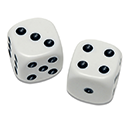#RandomizedTesting.Generators 2.7.8-beta-0002

This is a prerelease version of RandomizedTesting.Generators.
`Install-Package RandomizedTesting.Generators -Version 2.7.8-beta-0002`
`dotnet add package RandomizedTesting.Generators --version 2.7.8-beta-0002`
`<PackageReference Include="RandomizedTesting.Generators" Version="2.7.8-beta-0002" />`
For projects that support PackageReference, copy this XML node into the project file to reference the package.
`paket add RandomizedTesting.Generators --version 2.7.8-beta-0002`
`#r "nuget: RandomizedTesting.Generators, 2.7.8-beta-0002"`
#r directive can be used in F# Interactive, C# scripting and .NET Interactive. Copy this into the interactive tool or source code of the script to reference the package.
```// Install RandomizedTesting.Generators as a Cake Addin

// Install RandomizedTesting.Generators as a Cake Tool
#tool nuget:?package=RandomizedTesting.Generators&version=2.7.8-beta-0002&prerelease```

## Randomized TestingThis is a C# port of the only the generators from the Java randomizedtesting library.

In addition to the random generators, the `RandomExtensions` class contains several useful low-level extension methods for generating numbers, text (html-like, regex-like, unicode, realistic unicode, plain ASCII), random picks from collections, or random regular expression instances.

### Random Generator Examples

``````// Create a System.Random instance
Random random = new Random();

// Create a random bool
bool boolValue = random.NextBoolean();

// Create a random long
long longValue = random.NextInt64();

// Create a random long between 10 (inclusive) and 100 (inclusive)
long longRangeValue = random.NextInt64(minValue: 10, maxValue: 100);

// Create a random float
float floatValue = random.NextSingle();

// Create a random BigInteger (from System.Numerics)
BigInteger bigIntValue = random.NextBigInteger();

// Pick a random KeyValuePair from a generic dictionary
var dictionary = new Dictionary<string, string>
{
["one"] = "oneValue",
["two"] = "twoValue",
["three"] = "threeValue",
["four"] = "fourValue",
["five"] = "fiveValue",
};
KeyValuePair<string, string> kvp = random.NextFrom(dictionary);

// Pick a random element from an array
var array = new int[] { 1, 4, 7, 10, 14, 74, 136 };
var arrayElement = random.RandomFrom(array);

// Create a random string (plain ASCII)
string asciiValue = random.NextSimpleString(maxLength: 15);

// Create a random string with specific chars
string specValue = random.NextSimpleStringRange(minChar: 'A', maxChar: 'F', maxLength: 10);

// Create a random Unicode string (may contain surrogate pairs)
string unicodeValue = random.NextUnicodeString(maxLength: 20);

// Create a random realistic Unicode string (random characters picked from the same Unicode block)
string realUnicodeValue = random.NextRealisticUnicodeString(maxLength: 30);

// Fill part of a char[] with random Unicode characters
char[] chars = "The quick brown fox jumped over the lazy dog.".ToCharArray();
random.NextFixedLengthUnicodeString(chars, startIndex: 8, length: 20);

// Generate a random regex-like string
string regexLike = random.NextRegexishString(maxLength: 20);

// Generate a random HTML-like string
string htmlLike = random.NextHtmlishString(numElements: 144);

// Randomly recase a string
string toRecase = "This Is A Pascal Cased String";
string recased = random.NextStringRecasing(toRecase);

// Generate a random Regex that compiles
Regex pattern = random.NextRegex();
``````

### Saying Thanks

If you find this library to be useful, please star us on GitHub and consider a sponsorship so we can continue bringing you great free tools like this one. It really makes a big difference!• #### .NETFramework 4.5

• J2N (>= 2.0.0-beta-0017)
• #### .NETStandard 2.0

• J2N (>= 2.0.0-beta-0017)

### NuGet packages (2)

Showing the top 2 NuGet packages that depend on RandomizedTesting.Generators:

Lucene.Net.TestFramework

Framework for testing third-party code that uses the Lucene.Net API using NUnit.

RandomizedTesting

This is a package dependency that is not meant to be referenced directly. Please use https://www.nuget.org/packages/RandomizedTesting.Generators instead.

### GitHub repositories (1)

Showing the top 1 popular GitHub repositories that depend on RandomizedTesting.Generators:

Repository Stars
apache/lucenenet
Apache Lucene.NET